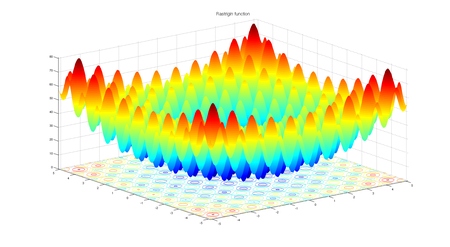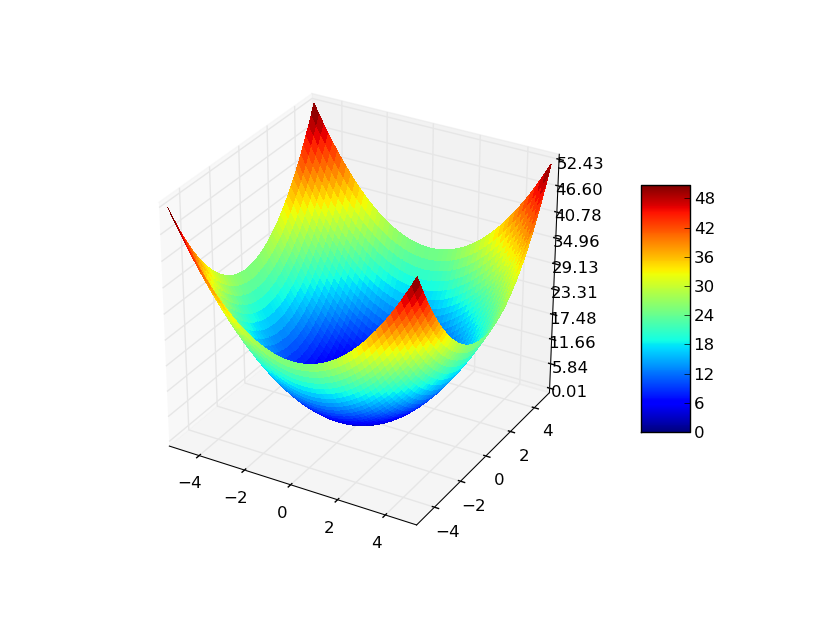Related Articles

# Implementation of Teaching Learning Based Optimization

• Last Updated : 18 Jul, 2021

Previous article Teaching Learning based Optimization (TLBO) talked about inspiration of teaching learning based optimization , it’s mathematical modelling and algorithm. In this article we will implement Teaching learning based optimization (TLBO) for two fitness functions 1) Rastrigin function    2) Sphere function.  The algorithm will run for a predefined number of maximum iterations and will try to find the minimum value of these fitness functions.

## Fitness function

### 1) Rastrigin function

Rastrigin function is a non-convex function and is often used as a performance test problem for optimization algorithms.

#### function equation:Fig1: Rastrigin function for 2 variables

For an optimization algorithm, rastrigin function is a very challenging one. Its complex behaviour cause optimization algorithms to often stuck at local minima. Having a lot of cosine oscillations on the plane introduces the complex behaviour to this function.

### 2) Sphere function

Sphere function is a standard function for evaluating the performance of an optimization algorithm.

#### function equation:Fig 2: Sphere function for 2 variables

## Choice of hyper-parameters

### Parameters of problem:

• Number of dimensions (d) = 3
• Lower bound (minx) = -10.0
• Upper bound (maxx) = 10.0

### Hyperparameters of the algorithm:

• Number of particles (N) = 50
• Maximum number of iterations (max_iter) = 100

### Inputs

• Fitness function
• Problem parameters ( mentioned above)
• Population size (N) and Maximum number of iterations  (max_iter)
• Algorithm Specific hyper parameters (None in teaching learning based optimization)

## Pseudocode

The pseudocode of the teaching-learning based optimization is already described in the previous article. Data structures to store students as well as data structure to store data specific to individual student were also discussed.

## Python3

 # python implementation of Teaching learning based optimization (TLBO)# minimizing rastrigin and sphere function  import randomimport math    # cos() for Rastriginimport copy    # array-copying convenienceimport sys     # max float    #-------fitness functions---------  # rastrigin functondef fitness_rastrigin(position):  fitness_value = 0.0  for i in range(len(position)):    xi = position[i]    fitness_value += (xi * xi) - (10 * math.cos(2 * math.pi * xi)) + 10  return fitness_value  #sphere functiondef fitness_sphere(position):    fitness_value = 0.0    for i in range(len(position)):        xi = position[i]        fitness_value += (xi*xi);    return fitness_value;#-------------------------  #Student classclass Student:  def __init__(self, fitness, dim, minx, maxx, seed):    self.rnd = random.Random(seed)      # a list of size dim     # with 0.0 as value of all the elements    self.position = [0.0 for i in range(dim)]      # loop dim times and randomly select value of decision var    # value should be in between minx and maxx    for i in range(dim):      self.position[i] = ((maxx - minx) *        self.rnd.random() + minx)      # compute the fitness of student    self.fitness = fitness(self.position)    # Teaching learning based optimizationdef tlbo(fitness, max_iter, n, dim, minx, maxx):  rnd = random.Random(0)    # create n random students  classroom = [Student(fitness, dim, minx, maxx, i) for i in range(n)]     # compute the value of best_position and best_fitness in the classroom  Xbest = [0.0 for i in range(dim)]  Fbest = sys.float_info.max            for i in range(n): # check each Student    if classroom[i].fitness < Fbest:      Fbest = classroom[i].fitness      Xbest = copy.copy(classroom[i].position)     # main loop of tlbo  Iter = 0  while Iter < max_iter:          # after every 10 iterations     # print iteration number and best fitness value so far    if Iter % 10 == 0 and Iter > 1:      print("Iter = " + str(Iter) + " best fitness = %.3f" % Fbest)      # for each student of classroom    for i in range(n):         ### Teaching phase of ith student        # compute the mean of all the students in the class      Xmean = [0.0 for i in range(dim)]      for k in range(n):          for j in range(dim):              Xmean[j]+= classroom[k].position[j]              for j in range(dim):          Xmean[j]/= n;              # initialize new solution      Xnew = [0.0 for i in range(dim)]        # teaching factor (TF)      # either 1 or 2 ( randomly chosen)      TF = random.randint(1, 3)        # best student of the class is teacher      Xteacher = Xbest        # compute new solution       for j in range(dim):          Xnew[j] = classroom[i].position[j] + rnd.random()*(Xteacher[j] - TF*Xmean[j])              # if Xnew < minx OR Xnew > maxx      # then clip it       for j in range(dim):          Xnew[j] = max(Xnew[j], minx)          Xnew[j] = min(Xnew[j], maxx)              # compute fitness of new solution      fnew = fitness(Xnew)        # if new solution is better than old       # replace old with new solution      if(fnew < classroom[i].fitness):          classroom[i].position = Xnew          classroom[i].fitness = fnew               # update best student      if(fnew < Fbest):          Fbest = fnew          Xbest = Xnew            ### learning phase of ith student        # randomly choose a solution from classroom      # chosen solution should not be ith student      p = random.randint(0, n-1)      while(p==i):          p = random.randint(0, n-1)              # partner solution      Xpartner = classroom[p]        Xnew = [0.0 for i in range(dim)]      if(classroom[i].fitness < Xpartner.fitness):          for j in range(dim):              Xnew[j] = classroom[i].position[j] + rnd.random()*(classroom[i].position[j] - Xpartner.position[j])      else:          for j in range(dim):              Xnew[j] = classroom[i].position[j] - rnd.random()*(classroom[i].position[j] - Xpartner.position[j])        # if Xnew < minx OR Xnew > maxx      # then clip it       for j in range(dim):          Xnew[j] = max(Xnew[j], minx)          Xnew[j] = min(Xnew[j], maxx)              # compute fitness of new solution      fnew = fitness(Xnew)        # if new solution is better than old       # replace old with new solution      if(fnew < classroom[i].fitness):          classroom[i].position = Xnew          classroom[i].fitness = fnew               # update best student      if(fnew < Fbest):          Fbest = fnew          Xbest = Xnew      Iter += 1  # end-while    # return best student from classroom  return Xbest# end pso    #----------------------------# Driver code for rastrigin function  print("\nBegin  teaching learning based optimization on rastrigin function\n")dim = 3fitness = fitness_rastrigin    print("Goal is to minimize Rastrigin's function in " + str(dim) + " variables")print("Function has known min = 0.0 at (", end="")for i in range(dim-1):  print("0, ", end="")print("0)")  num_particles = 50max_iter = 100  print("Setting num_particles = " + str(num_particles))print("Setting max_iter    = " + str(max_iter))print("\nStarting TLBO algorithm\n")      best_position = tlbo(fitness, max_iter, num_particles, dim, -10.0, 10.0)  print("\nTLBO completed\n")print("\nBest Student found:")print(["%.6f"%best_position[k] for k in range(dim)])fitness_value = fitness(best_position)print("fitness of best Student = %.6f" % fitness_value)  print("\nEnd TLBO for rastrigin function\n")    print()print()    # Driver code for Sphere function print("\nBegin teaching learning based optimization on sphere function\n")dim = 3fitness = fitness_sphere    print("Goal is to minimize sphere function in " + str(dim) + " variables")print("Function has known min = 0.0 at (", end="")for i in range(dim-1):  print("0, ", end="")print("0)")  num_particles = 50max_iter = 100  print("Setting num_particles = " + str(num_particles))print("Setting max_iter    = " + str(max_iter))print("\nStarting TLBO algorithm\n")      best_position = tlbo(fitness, max_iter, num_particles, dim, -10.0, 10.0)  print("\nTLBO completed\n")print("\nBest Student found:")print(["%.6f"%best_position[k] for k in range(dim)])fitness_value = fitness(best_position)print("fitness of best Student = %.6f" % fitness_value)  print("\nEnd TLBO for sphere function\n")

## Output

Begin  teaching learning based optimization on rastrigin function

Goal is to minimize Rastrigin's function in 3 variables
Function has known min = 0.0 at (0, 0, 0)
Setting num_particles = 50
Setting max_iter    = 100

Starting TLBO algorithm

Iter = 10 best fitness = 3.662
Iter = 20 best fitness = 0.389
Iter = 30 best fitness = 0.389
Iter = 40 best fitness = 0.389
Iter = 50 best fitness = 0.200
Iter = 60 best fitness = 0.132
Iter = 70 best fitness = 0.051
Iter = 80 best fitness = 0.003
Iter = 90 best fitness = 0.001

TLBO completed

Best Student found:
['0.000593', '-0.000040', '-0.000461']
fitness of best Student = 0.000112

End TLBO for rastrigin function

Begin teaching learning based optimization on sphere function

Goal is to minimize sphere function in 3 variables
Function has known min = 0.0 at (0, 0, 0)
Setting num_particles = 50
Setting max_iter    = 100

Starting TLBO algorithm

Iter = 10 best fitness = 0.009
Iter = 20 best fitness = 0.000
Iter = 30 best fitness = 0.000
Iter = 40 best fitness = 0.000
Iter = 50 best fitness = 0.000
Iter = 60 best fitness = 0.000
Iter = 70 best fitness = 0.000
Iter = 80 best fitness = 0.000
Iter = 90 best fitness = 0.000

TLBO completed

Best Student found:
['0.000000', '-0.000000', '-0.000000']
fitness of best Student = 0.000000

End TLBO for sphere function

### References

Research paper: R. V. Rao, V. J. Savsani & J. Balic (2012) Teaching–learning-based optimization algorithm for unconstrained and constrained real-parameter optimization problems, Engineering Optimization, 44:12, 1447-1462, DOI: 10.1080/0305215X.2011.652103

Inspiration of the implementation: https://www.mathworks.com/matlabcentral/fileexchange/65628-teaching-learning-based-optimization#:~:text=Teaching%20Learning%20Based%20Optimization%20is,teacher%20and%20the%20student%20phase.

Attention reader! Don’t stop learning now. Get hold of all the important Machine Learning Concepts with the Machine Learning Foundation Course at a student-friendly price and become industry ready.

My Personal Notes arrow_drop_up